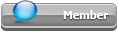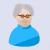## Featured Articles

Check out the latest featured articles.## File Library0# Propane Gas Volume To Liquid Volume Conversion

propane liquid conversion mass volume flowrate fuel

|

### #1james.corrobro@gmail.com

james.corrobro@gmail.com

Veteran Member

•• Members
•• 35 posts

Posted 26 March 2021 - 05:11 PM

hello all, i am trying to convert a gas flowrate of propane to equivalent liquid volume and mass.

My consumption of propane is at 0.5 mmscfd. I have tried to do following calculation by converting gas propane to mass and then using that mass and liquid density to convert to liquid volume,. Can any experts take a review and see if this calculation looks good. or if there is any mistake

Propane Consumption = 0.5 MMSCFD= 0.5 x 10^6 / 24 = 20833 scfh

This is at 100 psig and 65°F

I convert this standard flow to actual flow using ideal gas law

Tact = 65 F = 460 + 65 = 525°R

Pact = 100 psig = 100 + 14.7 = 114.7 psia

Tstd = 60 F = 460 + 60 = 520°R

Pstd = 14.7 psia

R = 10.73 ft3 psi / lbmol °R

Pact Vact / Tact = Pstd Vstd / Tstd

Vact = Pstd Vstd / Tstd x Tact/Pact

Vact = 14.7 x 20833 / 520 x 525/114.7

Vact = 2965 ACFH

Number of moles of Propane can be found by PV = nRT

n = PV / RT

n = 114.7 x 2695 / 10.73 x 525 = 54.86 lbmol/h

Propane Mol Wt = 44.1 lb/lbmol

Propane Mass Consumption = 54.86 x 44.1 lb/h = 2419 lb/h (gas mass = liquid mass; mass conservation)

Propane Liquid Density at ambient conditions (80F ) = 30.58 lb/ft3

Propane Liquid Volume Consumption = 2419/30.58 = 79 ft3/h

### #2Pilesar

Pilesar

Gold Member

•• Members
•• 949 posts

Posted 26 March 2021 - 09:55 PM

Your final answer is close enough for two significant figures. Close enough is good enough so I won't call out any 'mistakes' and instead just make a few comments on your calcs.

1) It seems unnecessarily confusing to use the actual gas flow conditions in your calcs to get the molar rate. Your flowrate is already standardized and scf converts directly to lbmol (379.5 scf/lbmol for std conditions of 1 atm, 60F.)
2) Liquid volume may not be so simple since ambient temperature is probably not a constant 80 F. The reference temperature for propane liquid volume is usually 60 F so when propane is bought or sold by the gallon, the actual volume is corrected for temperature. That may be important if you calculate a cost for the propane flow.
3) It is probable that your propane is not 100% C3H8 as assumed in your calcs which will add error into your molecular weight assumption. High grade HD5 Propane is spec'd to be minimum 90% propane.

Edited by Pilesar, 26 March 2021 - 10:18 PM.